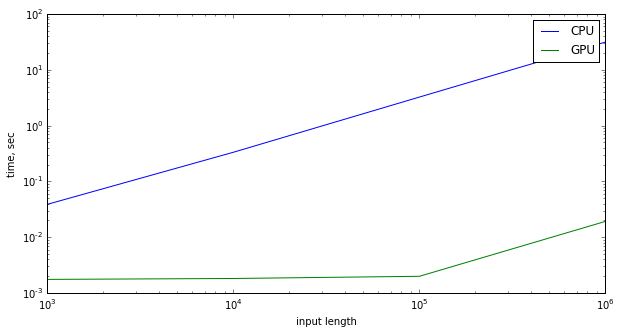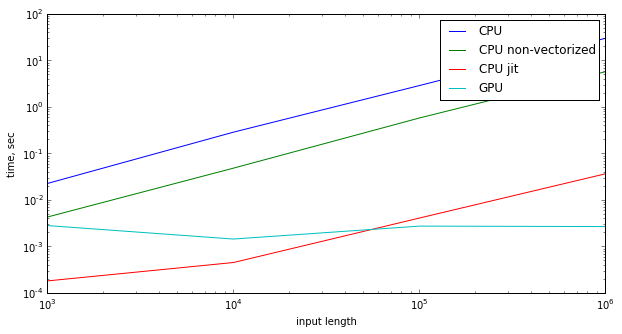# Extracting 1DLBP Pattern with Python, Numba & CUDA

By | July 14, 2015
Now that I have learned how to convert IPython notebooks to WordPress-able html, here is an old post revisited.

## Extracting a 1D Local Binary Pattern Histogram on NVIDIA GPU with CUDA and Numbapro¶

This was done for the Microsoft Malware competition on Kaggle. In this contest, a bunch of malware files needed to be classified in 9 categories. The files were presented in two sets, 10,868 each:

• text (disassembly of the malware)
• binary (actual binaries minus PE header to render them harmless)

I chose the 1DLBP algorithm described in the linked paper to extract (hopefully meaningful) features from each binary file. These features, in combination with some text-based features extracted from the disassemblies, proved to be quite effective in training an accurate classifier

### Prerequisits¶

• basic knowledge of CUDA programming (kernels, grid, blocks, threads, etc)
• numba
In :
from numba import *
from timeit import default_timer as timer

import numpy as np
import matplotlib.pylab as plt


### Algorithm in Brief¶

Since the paper (above) does a great job explaining the algorithm, I will only describe it very briefly.

For every byte (b) in the image, we look at 4 (or any neighborhood) of bytes immediately to the “left” and at 4 bytes immediately to the “right” of the current byte.

We now have 8 selected bytes, call them c[], where size(c) = 8. Each of the elements of array c is converted into a binary digit, based on the following rule:

In :
c = np.random.randint(0, 256, size=8) #neighborhood bytes to the left and to the right contain these values
b = np.random.randint(256) # center of the neighborhood
digits = np.array([0 if d < b else 1 for d in c]) # this is the number that represents the pattern
print "Center: ", b
print "Bytes around it: ", c
print "Pattern: ", digits

# In order to compute the actual pattern, we just mulitply each of the digits by a power of two and add them up
powers = 1 << np.arange(0, 8)
print "As number base 10: ", np.dot(powers, digits.T)

Center:  2
Bytes around it:  [252 156   3  24 120  25  85  60]
Pattern:  [1 1 1 1 1 1 1 1]
As number base 10:  255


Which is actually a non-negative integer less than $2^8$.

Then a histogram of these values is built. It has the size of 256 ints and in training experiments proves to be much more effective than a simple byte histogram.

The functions below translate this description into Python:

In :
def extract_1dlbp_cpu(input, neighborhood, p):
"""
Extract the 1d lbp pattern on CPU
"""
res = np.zeros(1 << (2 * neighborhood))
for i in range(neighborhood, len(input) - neighborhood):
left = input[i - neighborhood : i]
right = input[i + 1 : i + neighborhood + 1]
both = np.r_[left, right]
res[np.sum(p [both >= input[i]])] += 1
return res


With numba, this can be expressed on the GPU using CUDA. If you have numbapro, CUDA support can be validated:

In :
import numbapro
numbapro.check_cuda()

------------------------------libraries detection-------------------------------
Finding cublas
located at C:\Anaconda\DLLs\cublas64_60.dll
trying to open library...	ok
Finding cusparse
located at C:\Anaconda\DLLs\cusparse64_60.dll
trying to open library...	ok
Finding cufft
located at C:\Anaconda\DLLs\cufft64_60.dll
trying to open library...	ok
Finding curand
located at C:\Anaconda\DLLs\curand64_60.dll
trying to open library...	ok
Finding nvvm
located at C:\Anaconda\DLLs\nvvm64_20_0.dll
trying to open library...	ok
finding libdevice for compute_20...	ok
finding libdevice for compute_30...	ok
finding libdevice for compute_35...	ok
-------------------------------hardware detection-------------------------------
Found 1 CUDA devices
id 0       GeForce GTX TITAN                              [SUPPORTED]
compute capability: 3.5
pci device id: 0
pci bus id: 2
Summary:
1/1 devices are supported
PASSED

Out:
True

I am working with GTX Titan, 14 SMs (14 * 192 = 2688 SPs), 6 Gb RAM. This used to be cool a year and a half ago, but a lot has happened since then.

### The Kernel¶

In :
@cuda.jit('void(uint8[:], int32, int32[:], int32[:])')
def lbp_kernel(input, neighborhood, powers, h):
i = cuda.grid(1)
r = 0
if i < input.shape - 2 * neighborhood:
i += neighborhood
for j in range(i - neighborhood, i):
if input[j] >= input[i]:
r += powers[j - i + neighborhood]

for j in range(i + 1, i + neighborhood + 1):
if input[j] >= input[i]:
r += powers[j - i + neighborhood - 1]



This looks like a regular CUDA kernel. It almost looksy “C++y”. Each thread is tasked with computing the pattern (“digits” above) around one single element of the original input array. We compute the pattern, then wait for all of the threads in the block to finish and update the histogram with their results.

Note: implementation of histogramming using atomics is the most straightforward, but not the most efficient one. For our purposes it will do just fine.

### Calling the Kernel¶

That is pretty standard: allocate memory and move it to the GPU.

In :
def extract_1dlbp_gpu(input, neighborhood, d_powers):
'''
input - the input array
neighborhood - size of the neighborhood
d_powers - device address of the powers of two constants used to compute the final pattern
'''
d_input = cuda.to_device(input)

hist = np.zeros(2 ** (2 * neighborhood), dtype='int32')
gridDim = (len(input) - 2 * neighborhood + blockDim) / blockDim

d_hist = cuda.to_device(hist)

lbp_kernel[gridDim, blockDim](d_input, neighborhood, d_powers, d_hist)
d_hist.to_host()
return hist


### Testing¶

Running comparison for arrays of several lengths.

In :
X = np.arange(3, 7)
X = 10 ** X
neighborhood = 4

cpu_times = np.zeros(X.shape)
gpu_times = np.zeros(X.shape)

p = 1 << np.array(range(0, 2 * neighborhood), dtype='int32')
d_powers = cuda.to_device(p)

for i, x in enumerate(X):
input = np.random.randint(0, 256, size = x).astype(np.uint8)
start = timer()
h_cpu = extract_1dlbp_cpu(input, neighborhood, p)
cpu_times[i] = timer() - start
print "Finished on CPU: length: {0}, time: {1:3.4f}s".format(x, cpu_times[i])

start = timer()
h_gpu = extract_1dlbp_gpu(input, neighborhood, d_powers)
gpu_times[i] = timer() - start
print "Finished on GPU: length: {0}, time: {1:3.4f}s".format(x, gpu_times[i])
print "All h_cpu == h_gpu: ", (h_cpu == h_gpu).all()

Finished on CPU: length: 1000, time: 0.0331s
Finished on GPU: length: 1000, time: 0.0018s
All h_cpu == h_gpu:  True
Finished on CPU: length: 10000, time: 0.3532s
Finished on GPU: length: 10000, time: 0.0014s
All h_cpu == h_gpu:  True
Finished on CPU: length: 100000, time: 3.1897s
Finished on GPU: length: 100000, time: 0.0023s
All h_cpu == h_gpu:  True
Finished on CPU: length: 1000000, time: 30.9789s
Finished on GPU: length: 1000000, time: 0.0107s
All h_cpu == h_gpu:  True


### Charting¶

Here both axes are log-scaled.

In :
f = plt.figure(figsize=(10, 5))

plt.plot(X, cpu_times, label = "CPU")
plt.plot(X, gpu_times, label = "GPU")
plt.xlabel('input length')
plt.ylabel('time, sec')
plt.yscale('log')
plt.xscale('log')
plt.legend()
plt.show()### Improvements¶

The above results are a bit extreme and very boring. GPU is faster than CPU, however, 4 orders of magnitue?! Can we do better on CPU? Obviously the slowdown is due to Python complexity: array manipulations we are using for once… Here are things to try:

• Mimic CUDA kernel on CPU:
In :
def extract_1dlbp_gpu_debug(input, neighborhood, powers, res):
gridDim = (len(input) - 2 * neighborhood + blockDim) / blockDim

for block in range(0, gridDim):
r = 0
i = blockDim * block + thread
if i < input.shape - 2 * neighborhood:
i += neighborhood
for j in range(i - neighborhood, i):
if input[j] >= input[i]:
r += powers[j - i + neighborhood]

for j in range(i + 1, i + neighborhood + 1):
if input[j] >= input[i]:
r += powers[j - i + neighborhood - 1]

res[r] += 1
return res


A good thing, too, because this is actually a way to debug a CUDA kernel in Python

• Use numba to compile this simple code to something non-Python. For this we just decorate the definition like so:
In :
@jit("int32[:](uint8[:], int64, int32[:], int32[:])", nopython=True)
def extract_1dlbp_cpu_jit(input, neighborhood, powers, res):
gridDim = (len(input) - 2 * neighborhood + blockDim) / blockDim

for block in range(0, gridDim):
r = 0
i = blockDim * block + thread
if i < input.shape - 2 * neighborhood:
i += neighborhood
for j in range(i - neighborhood, i):
if input[j] >= input[i]:
r += powers[j - i + neighborhood]

for j in range(i + 1, i + neighborhood + 1):
if input[j] >= input[i]:
r += powers[j - i + neighborhood - 1]

res[r] += 1
return res


Now we modify the test:

In :
X = np.arange(3, 7)
X = 10 ** X
neighborhood = 4

cpu_times = np.zeros(X.shape)
cpu_times_simple = cpu_times.copy()
cpu_times_jit = cpu_times.copy()
gpu_times = np.zeros(X.shape)

p = 1 << np.array(range(0, 2 * neighborhood), dtype='int32')
d_powers = cuda.to_device(p)

for i, x in enumerate(X):
input = np.random.randint(0, 256, size = x).astype(np.uint8)

print "Length: {0}".format(x)
print "--------------"

start = timer()
h_cpu = extract_1dlbp_cpu(input, neighborhood, p)
cpu_times[i] = timer() - start
print "Finished on CPU: time: {0:3.5f}s".format(cpu_times[i])

res = np.zeros(1 << (2 * neighborhood), dtype='int32')
start = timer()
h_cpu_simple = extract_1dlbp_gpu_debug(input, neighborhood, p, res)
cpu_times_simple[i] = timer() - start
print "Finished on CPU (simple): time: {0:3.5f}s".format(cpu_times_simple[i])

res = np.zeros(1 << (2 * neighborhood), dtype='int32')
start = timer()
h_cpu_jit = extract_1dlbp_cpu_jit(input, neighborhood, p, res)
cpu_times_jit[i] = timer() - start
print "Finished on CPU (numba: jit): time: {0:3.5f}s".format(cpu_times_jit[i])

start = timer()
h_gpu = extract_1dlbp_gpu(input, neighborhood, d_powers)
gpu_times[i] = timer() - start
print "Finished on GPU: time: {0:3.5f}s".format(gpu_times[i])
print "All h_cpu == h_gpu: ", (h_cpu_jit == h_gpu).all() and (h_cpu_simple == h_cpu_jit).all() and (h_cpu == h_cpu_jit).all()
print ''

f = plt.figure(figsize=(10, 5))

plt.plot(X, cpu_times, label = "CPU")
plt.plot(X, cpu_times_simple, label = "CPU non-vectorized")
plt.plot(X, cpu_times_jit, label = "CPU jit")
plt.plot(X, gpu_times, label = "GPU")
plt.yscale('log')
plt.xscale('log')
plt.xlabel('input length')
plt.ylabel('time, sec')
plt.legend()
plt.show()

Length: 1000
--------------
Finished on CPU: time: 0.02263s
Finished on CPU (simple): time: 0.00430s
Finished on CPU (numba: jit): time: 0.00018s
Finished on GPU: time: 0.00281s
All h_cpu == h_gpu:  True

Length: 10000
--------------
Finished on CPU: time: 0.28701s
Finished on CPU (simple): time: 0.04830s
Finished on CPU (numba: jit): time: 0.00045s
Finished on GPU: time: 0.00144s
All h_cpu == h_gpu:  True

Length: 100000
--------------
Finished on CPU: time: 2.88549s
Finished on CPU (simple): time: 0.57943s
Finished on CPU (numba: jit): time: 0.00408s
Finished on GPU: time: 0.00274s
All h_cpu == h_gpu:  True

Length: 1000000
--------------
Finished on CPU: time: 29.97346s
Finished on CPU (simple): time: 5.73384s
Finished on CPU (numba: jit): time: 0.03651s
Finished on GPU: time: 0.00268s
All h_cpu == h_gpu:  TrueNow we are cooking! This grapph is much more interesting than the one above. GPU is still the winner, but, as expected, the investment of moving things between RAM and GPU memory pays off once the size of our data is sufficiently large. The GPU doesn’t get out of bed for anything smaller than about $10^5$. For anything else, if perf is crucial (as it very often is), we are better off writing it in a simple way and jitting. In other words: the best thing is not to write it in Python, but to do it in C++ from the get-go instead. Which appears to be a foregone conclusion, of course.